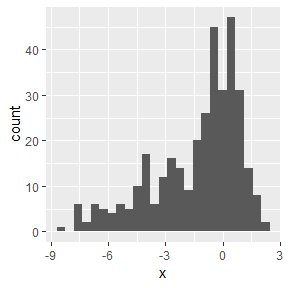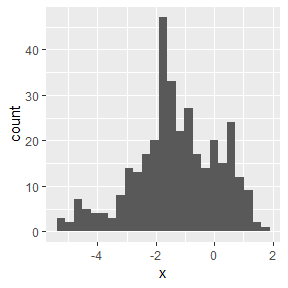# nonet_ensemble regression with nonet_plot

### nonet provides ensemble capabilities for regression problems.

Below example shows the step by step implementation of nonet_ensemble and nonet_plot functions in the context of regression. We have used Bank Note authentication data set to predict the output class variable using linear regression model. Predictions from first linear regression model and second linear regression model are being used as inputs to the nonet_ensemble in the list form.

Let’s start:

#### Load the required libraries

library(caret)
library(ggplot2)
library(nonet)

#### Load the banknote_authentication dataset and explore it.

dataframe <- data.frame(banknote_authentication)
head(dataframe)
##   variance skewness curtosis  entropy class
## 1  3.62160   8.6661  -2.8073 -0.44699     0
## 2  4.54590   8.1674  -2.4586 -1.46210     0
## 3  3.86600  -2.6383   1.9242  0.10645     0
## 4  3.45660   9.5228  -4.0112 -3.59440     0
## 5  0.32924  -4.4552   4.5718 -0.98880     0
## 6  4.36840   9.6718  -3.9606 -3.16250     0

### First Linear Regression Model

index <- createDataPartition(dataframe$class, p=0.75, list=FALSE) trainSet <- dataframe[ index,] testSet <- dataframe[-index,] ### Feature selection using rfe in caret control <- rfeControl(functions = rfFuncs, method = "repeatedcv", repeats = 3, verbose = FALSE) outcomeName <- 'entropy' predictors <- c("variance", "skewness", "class") #### Model Training banknote_lm_first <- train(trainSet[,predictors],trainSet[,outcomeName],method='lm') #### Predictions on testSet predictions_lm_first <- predict.train(object=banknote_lm_first, testSet[,predictors]) ### Second Linear Regression Model index <- createDataPartition(dataframe$class, p=0.75, list=FALSE)
trainSet <- dataframe[ index,]
testSet <- dataframe[-index,]

### Feature selection using rfe in caret

control <- rfeControl(functions = rfFuncs,
method = "repeatedcv",
repeats = 3,
verbose = FALSE)
outcomeName <- 'entropy'
predictors <- c("curtosis", "skewness", "class")

### Model Training

banknote_lm_second <- train(trainSet[,predictors],trainSet[,outcomeName],method='lm')

### Predictions on testSet

predictions_lm_second <- predict.train(object=banknote_lm_second, testSet[,predictors])

#### Create the stack of predictions

Stack_object <- list(predictions_lm_first, predictions_lm_second)

#### Applying naming to the Stack_object

names(Stack_object) <- c("lm_first", "lm_second")

#### nonet_ensemble

Now we need to apply the nonet_ensemble method by supplying list object and best model name as input. Note that We have not provided training or test outcome labels to compute the weights in the weighted average ensemble method, which is being used inside the none_ensemble. Thus it uses best models prediction to compute the weights in the weighted average ensemble.

prediction_nonet <- nonet_ensemble(Stack_object, "lm_first")

#### Creating the dataframe of nonet predictions and actual testSet labels to compute the accuracy

Actual_Pred <- data.frame(cbind(actuals = testSet[,outcomeName], predictions = prediction_nonet))
head(Actual_Pred)
##     actuals predictions
## 1  -0.44699   -1.869567
## 17  0.58619    1.340655
## 21 -0.48708   -1.994411
## 25 -3.74830   -1.780209
## 26 -2.87150   -1.227252
## 28 -3.74050   -3.537978

#### Evaluation Matrix

accuracy <- cor(Actual_Pred)
accuracy
##                actuals predictions
## actuals     1.00000000  0.09811443
## predictions 0.09811443  1.00000000

#### Result Plotting: nonet_plot

Results can be plotted using the nonet_plot function. nonet_plot is being designed to provided different plot_type options to the user so that one can plot different visualization based on their needs.

##### nonet_plot in histogram for the Actual labels in the testSet
plot_first <- nonet_plot(Actual_Pred$actuals, Actual_Pred$predictions, Actual_Pred, plot_type = "hist")
plot_first##### nonet_plot in histogram for the nonet_ensemble predictions
plot_second <- nonet_plot(Actual_Pred$predictions, Actual_Pred$actuals, Actual_Pred, plot_type = "hist")
plot_second### Conclusion

Above it can be seen that nonet_ensemble and nonet_plot can serve in a way that one do not need to worry about the outcome variables labels to compute the weights of weighted average ensemble solution.